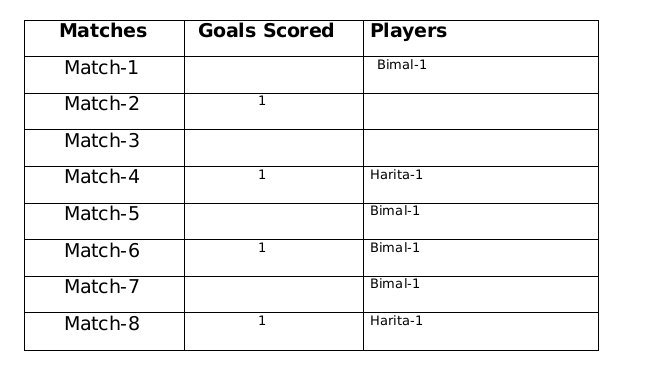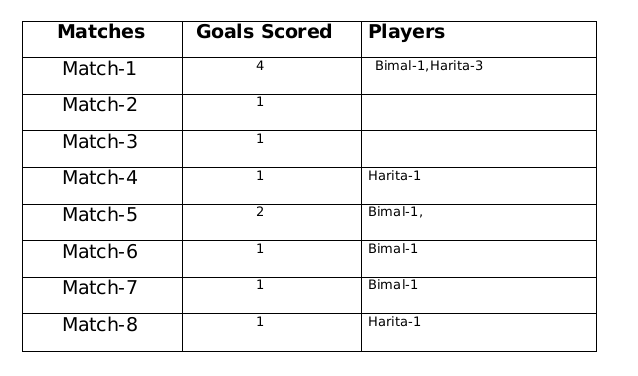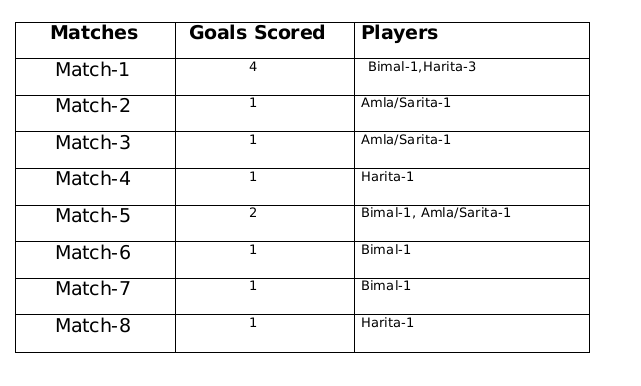### CAT 2022 Question Paper (Slot 1) Question 26

Instructions

The following facts are known about the goals scored by these four players only. All the questions refer only to the goals scored by these four players.

The management of a university hockey team was evaluating performance of four women players - Amla, Bimla, Harita and Sarita for their possible selection in the university team for next year. For this purpose, the management was looking at the number of goals scored by them in the past 8 matches, numbered 1 through 8. The four players together had scored a total of 12 goals in these matches. In the 8 matches, each of them had scored at least one goal. No two players had scored the same total number of goals.

1. Only one goal was scored in every even numbered match.
2. Harita scored more goals than Bimla.
3. The highest goal scorer scored goals in exactly 3 matches including Match 4 and Match 8.
4. Bimla scored a goal in Match 1 and one each in three other consecutive matches.
5. An equal number of goals were scored in Match 3 and Match 7, which was different from the number of goals scored in either Match 1 or Match 5.
6. The match in which the highest number of goals was scored was unique and it was not Match 5.

Question 26

# Which of the following is the correct sequence of goals scored in matches 1, 3, 5 and 7?

Solution

A total of 12 goals were scored in 8 matches and each player scored atleast one goal and no of goals scored by each one of them is distinct so the possible number of goals scored by the players can be (1,2,3,6) or (1,2,4,5).

From statement 4 we know that Bimal scored 4 goals and since Harita scored more goals than Bimal so we can say that Harita scored 5 goals and the only case possible for total goals scored by each of the players is (1,2,4,5).

Now using statement 1, statement 3 and statement 4 we can say that the three consecutive matches in which Bimal scored will be 5th, 6th and 7th matches as Harita scored in 4th and 8th matches and we get the following table:From statement 5 and 6, we can conclude that the highest number of goals were scored in Match 1.

Let the no. of goals scored in 3rd and 7th match be a each and no. of goals scored in 1st and 5th match be b and c respectively.

Therefore, 2a+b+c= 8

If a=1, then b+c=6 therefore possible solutions for b and c will be 2 and 4 only

If a=2, then b+c=4 therefore possible solution for b and c will be 1 and 3 only but since highest goals scored is in Match 1 so then no. of goals scored in match 1 must be 3 and Harita must have scored 3 goals in match 1 as Harita scored 5 goals in exactly 3 matches. Therefore, we can see this is not possible because then the no. of goals scored in Match 1 becomes 4.

Therefore the only possible solution is a=1, b=4 and c=2The remaining 3 goals were scored in the match 2, 3 and 5 by Amla and Sarita in some order.### View Video Solution

• All Quant CAT Formulas and shortcuts PDF
• 30+ CAT previous papers with solutions PDF

##### shubh g

7 months ago

sir,
doesn't either and or means that the score is either different for either 1 or 5 (one of them)?

OR